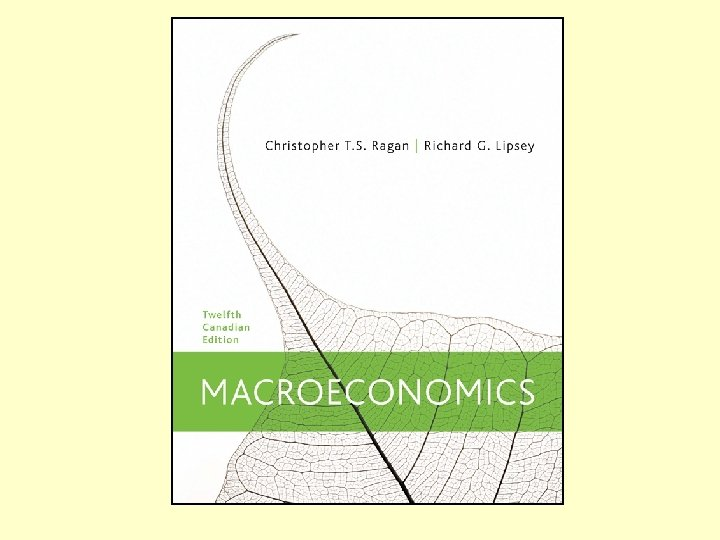Скачать презентацию Chapter 28 Money Interest Rates and Economic Activity

6ce18bd42ede155d0b54f4d3c15c4d96.ppt

• Количество слайдов: 63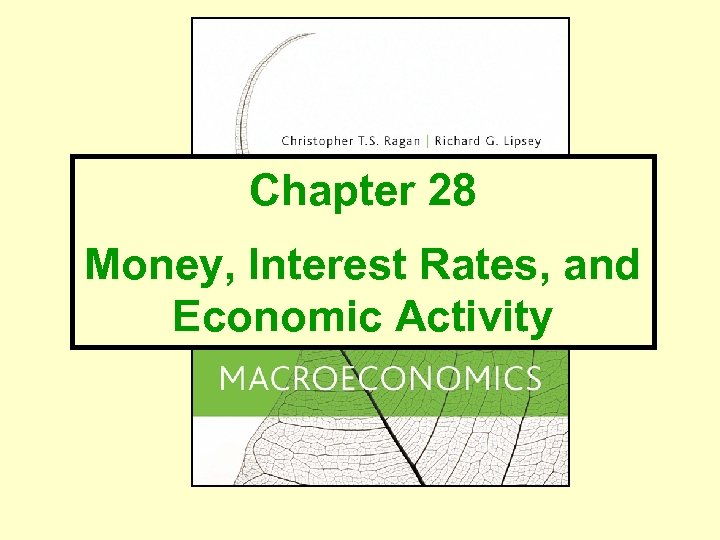Chapter 28 Money, Interest Rates, and Economic Activity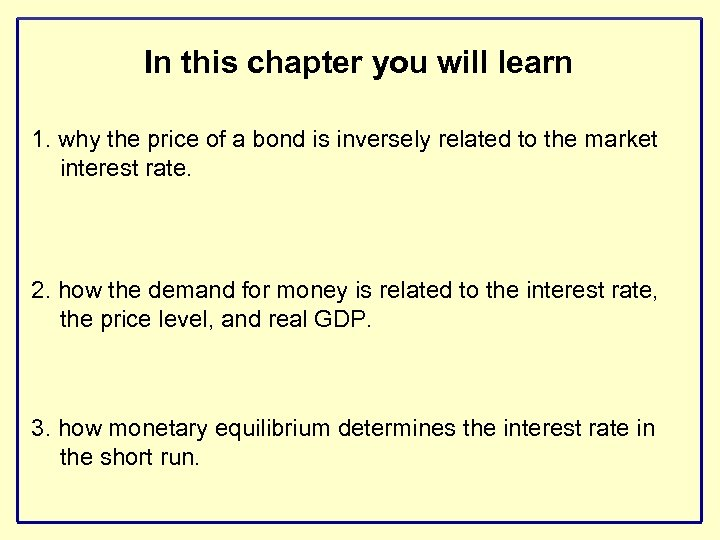In this chapter you will learn 1. why the price of a bond is inversely related to the market interest rate. 2. how the demand for money is related to the interest rate, the price level, and real GDP. 3. how monetary equilibrium determines the interest rate in the short run.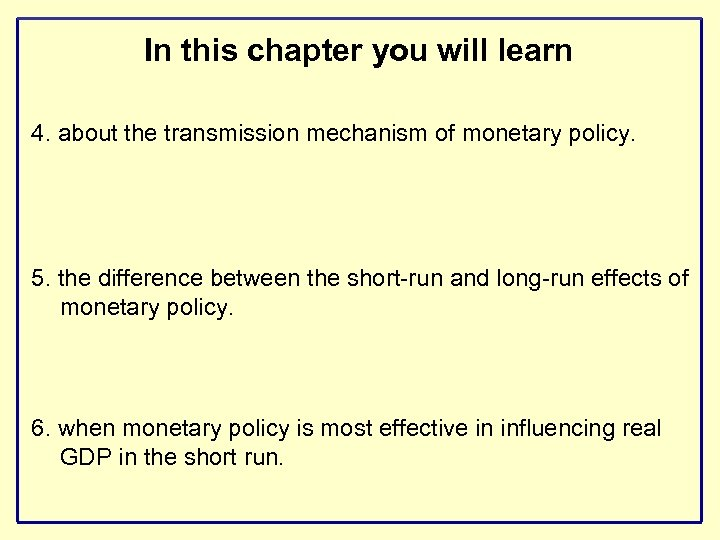In this chapter you will learn 4. about the transmission mechanism of monetary policy. 5. the difference between the short-run and long-run effects of monetary policy. 6. when monetary policy is most effective in influencing real GDP in the short run.For simplicity, we assume that people have two types of financial assets: - money (earns no interest) - bonds (earn interest)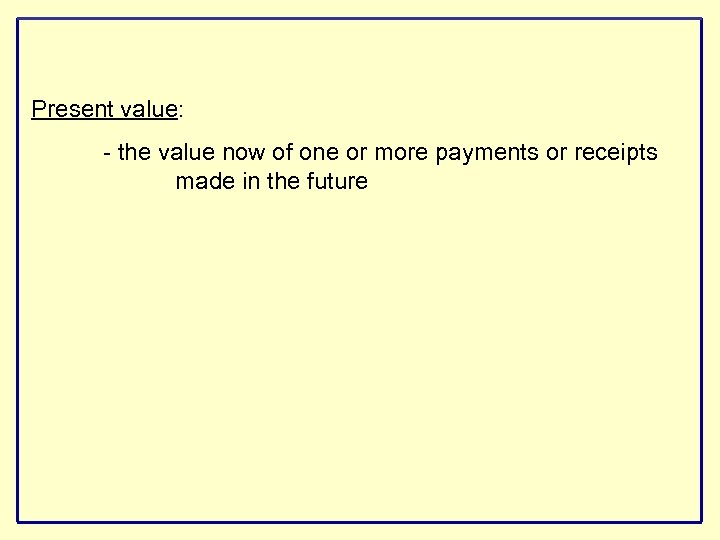Present value: - the value now of one or more payments or receipts made in the futureExamples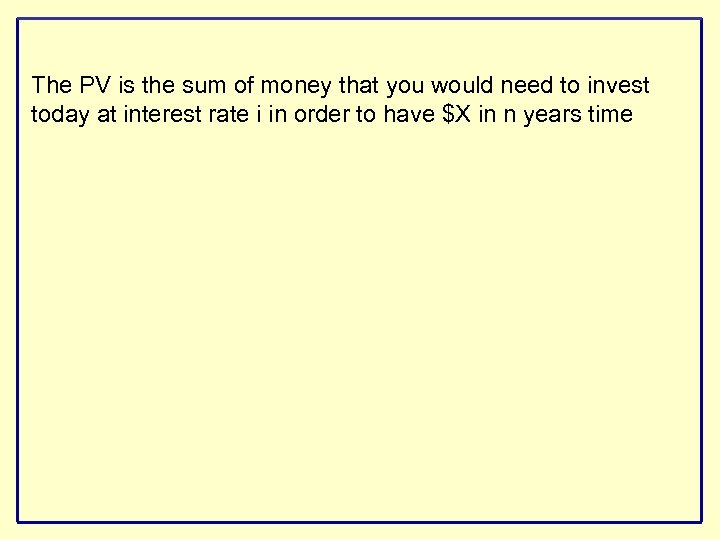The PV is the sum of money that you would need to invest today at interest rate i in order to have \$X in n years timeExamplesThe PV is the sum of money that you would need to invest today at interest rate i in order to have \$X in n years timeOPTIONALOPTIONAL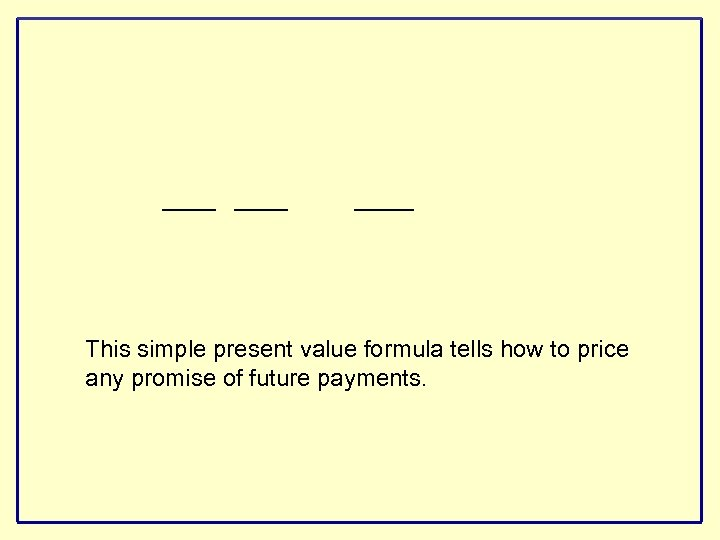This simple present value formula tells how to price any promise of future payments.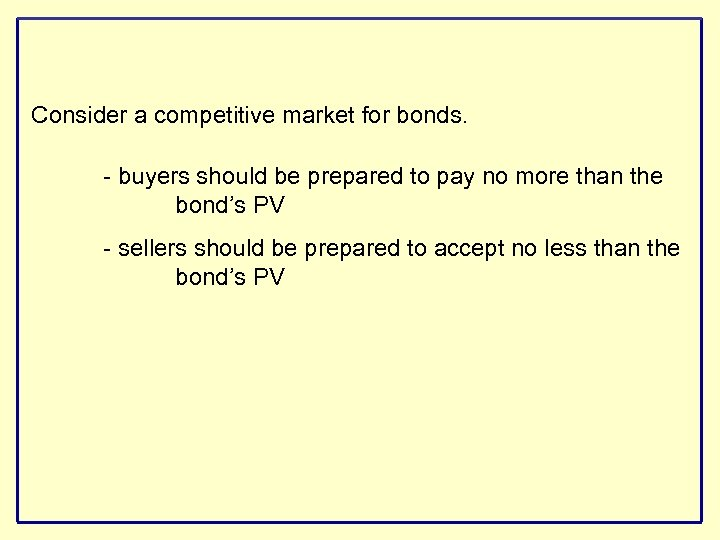Consider a competitive market for bonds. - buyers should be prepared to pay no more than the bond’s PV - sellers should be prepared to accept no less than the bond’s PV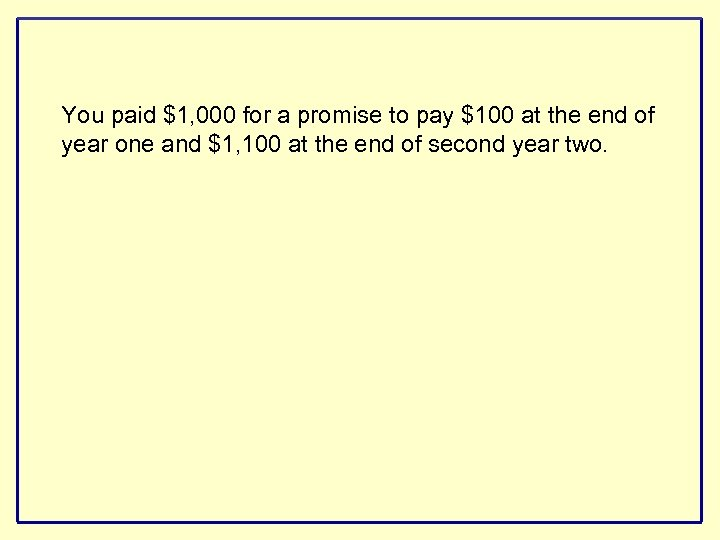You paid \$1, 000 for a promise to pay \$100 at the end of year one and \$1, 100 at the end of second year two.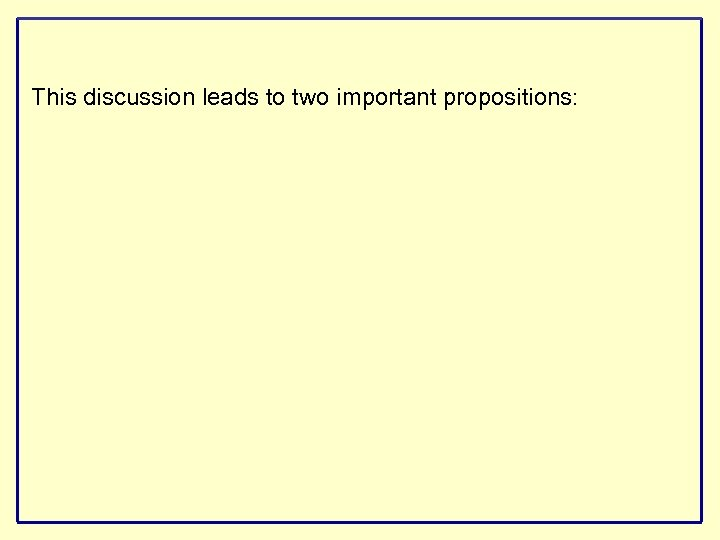This discussion leads to two important propositions: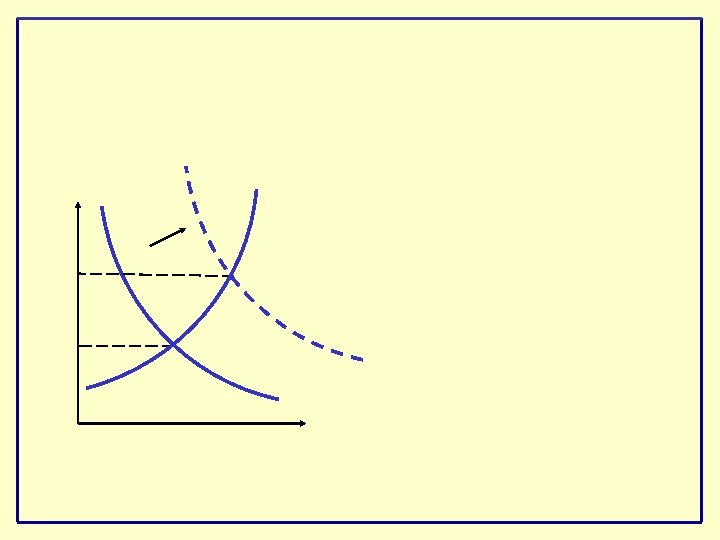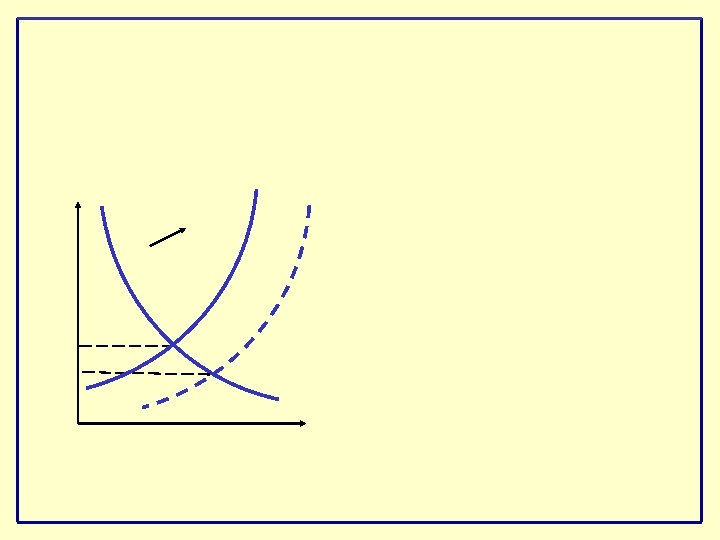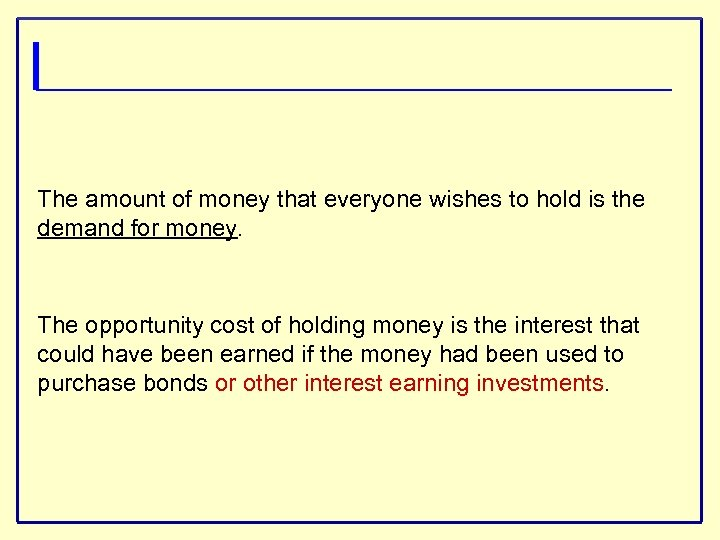The amount of money that everyone wishes to hold is the demand for money. The opportunity cost of holding money is the interest that could have been earned if the money had been used to purchase bonds or other interest earning investments.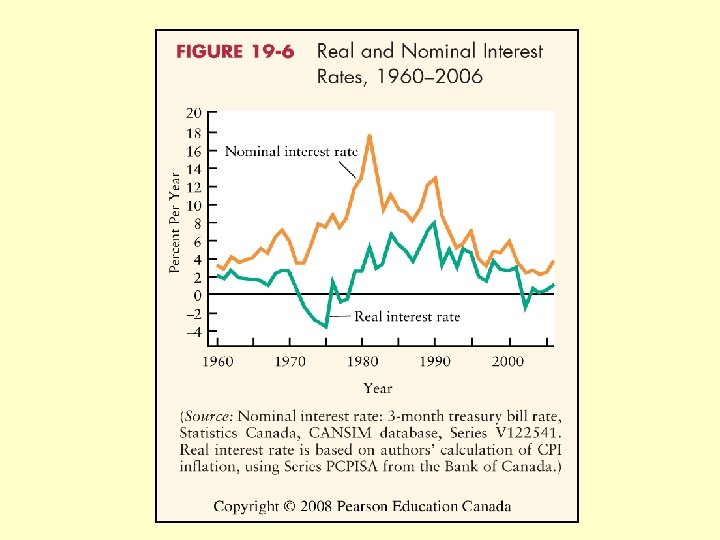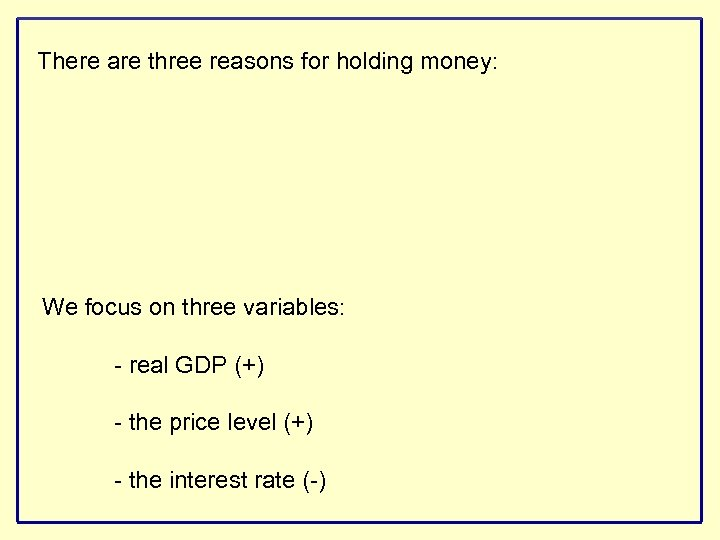There are three reasons for holding money: We focus on three variables: - real GDP (+) - the price level (+) - the interest rate (-)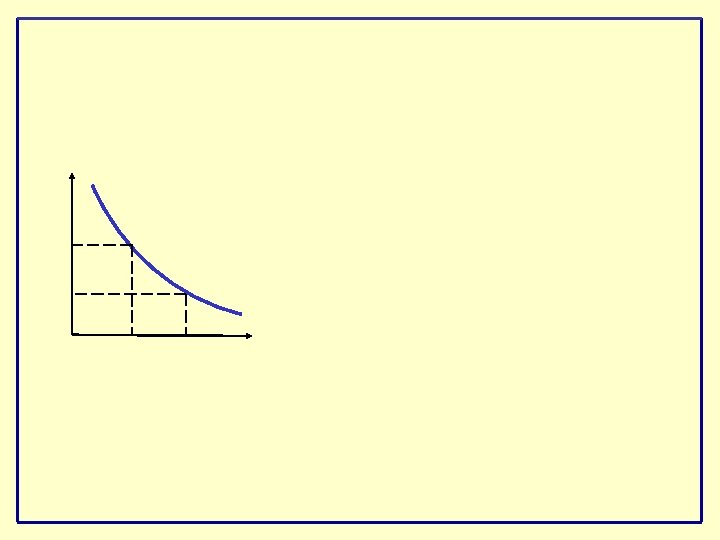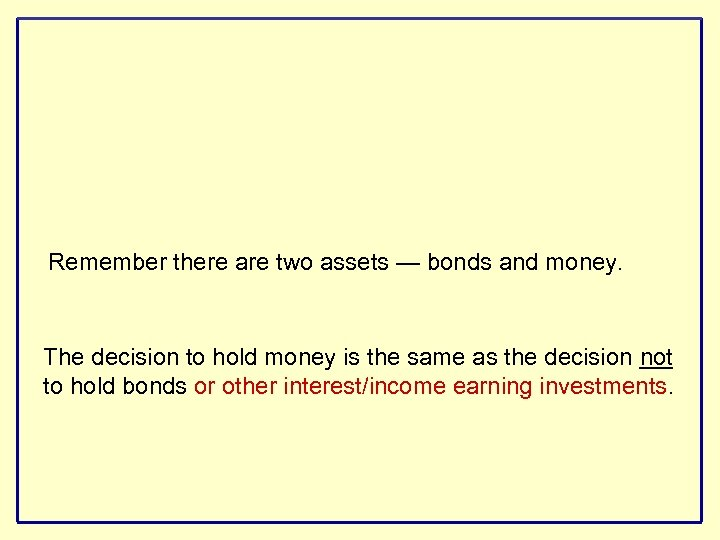Remember there are two assets — bonds and money. The decision to hold money is the same as the decision not to hold bonds or other interest/income earning investments.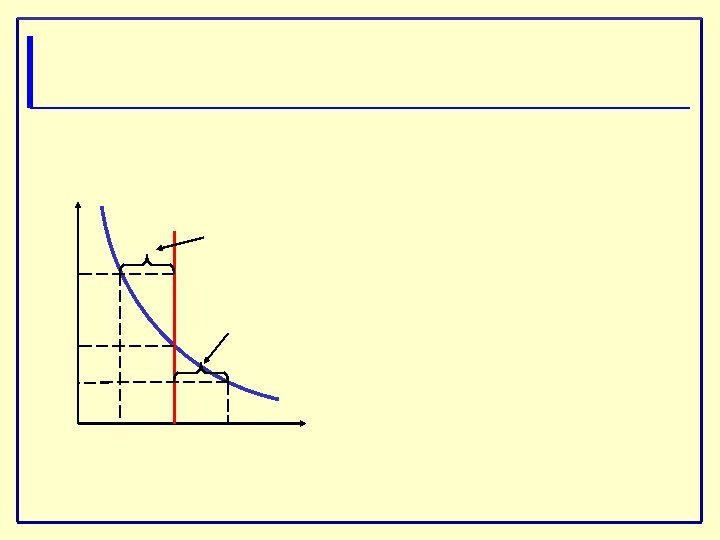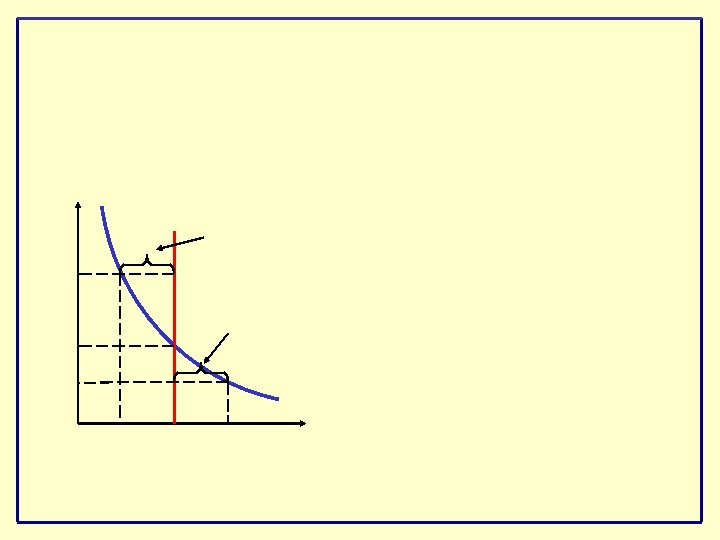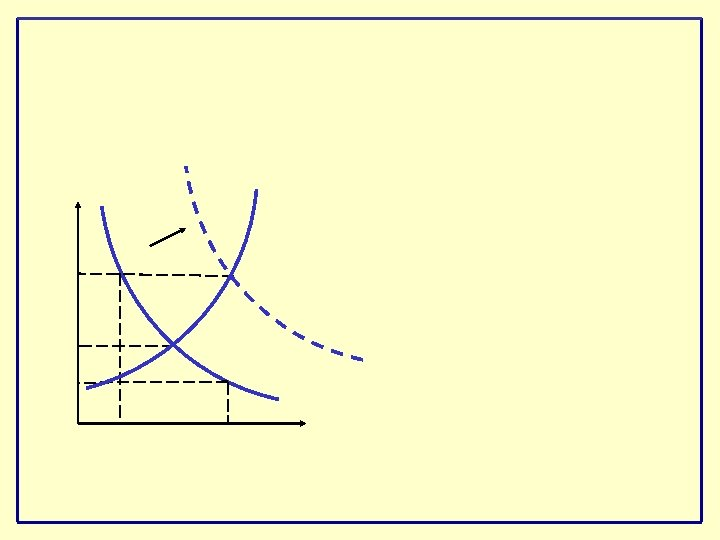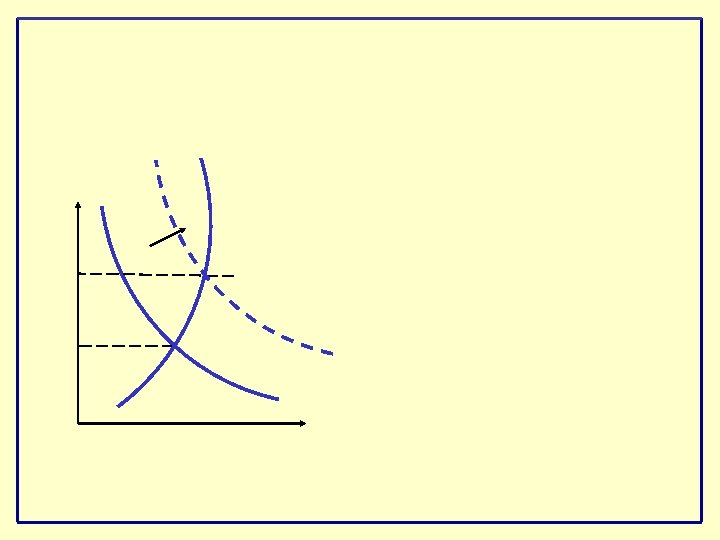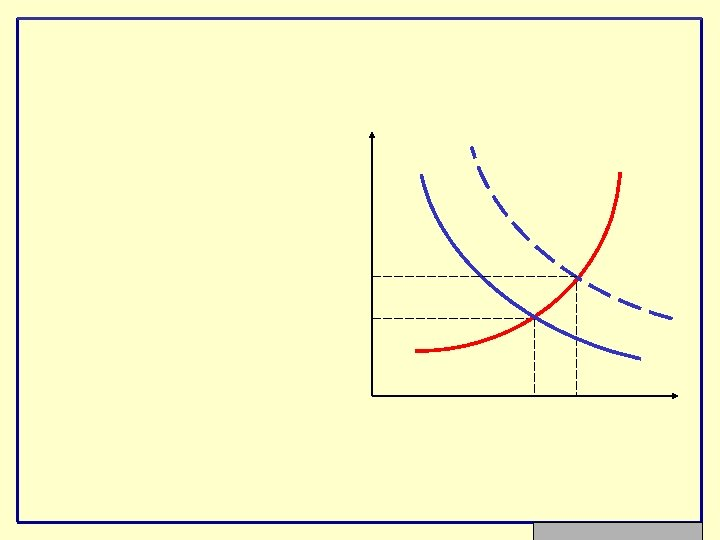Three stages: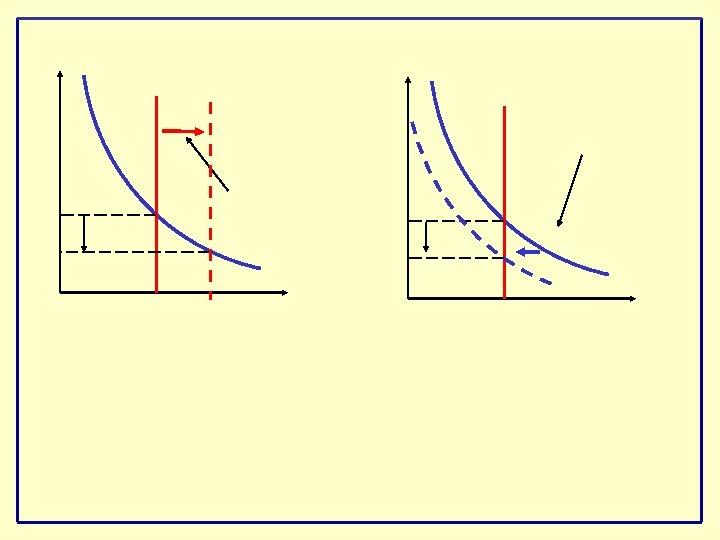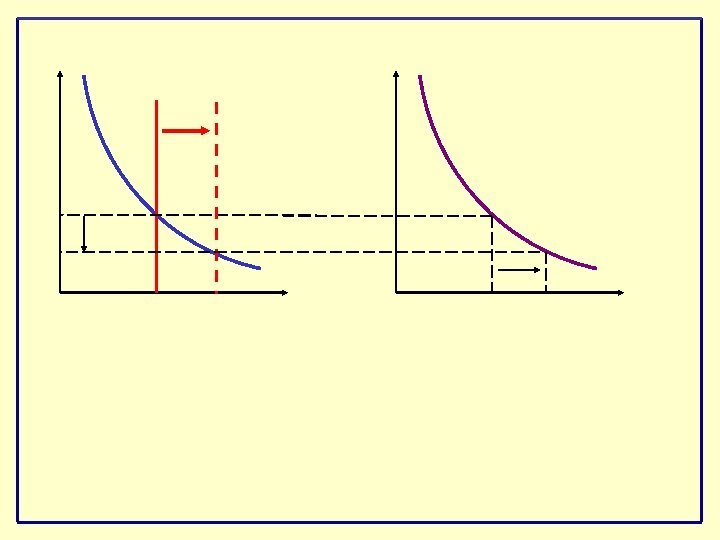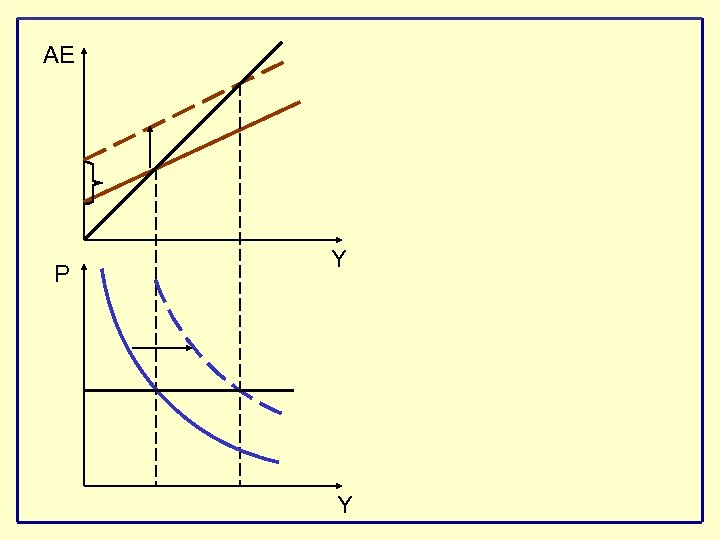AE P Y Y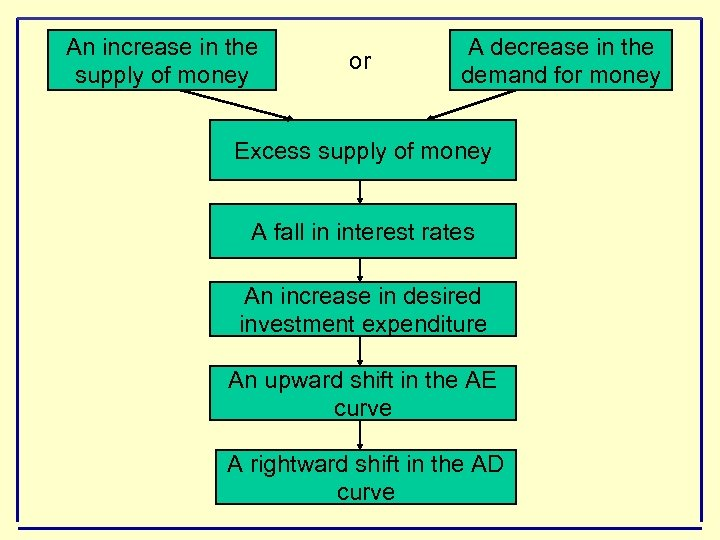An increase in the supply of money or A decrease in the demand for money Excess supply of money A fall in interest rates An increase in desired investment expenditure An upward shift in the AE curve A rightward shift in the AD curve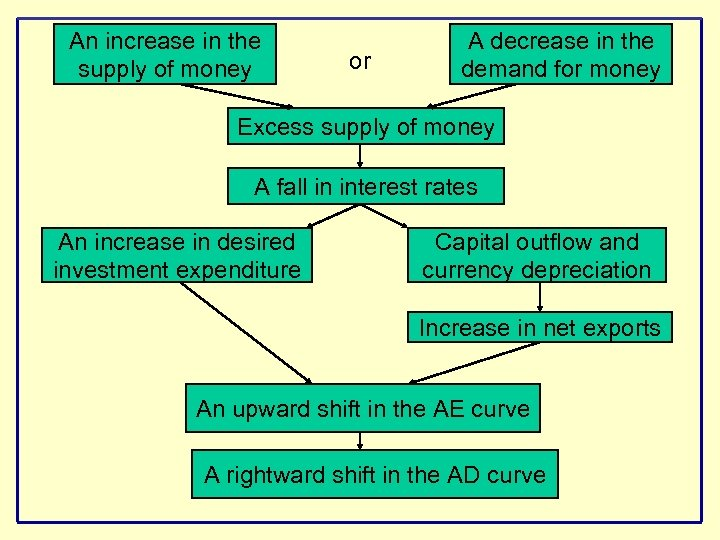An increase in the supply of money or A decrease in the demand for money Excess supply of money A fall in interest rates An increase in desired investment expenditure Capital outflow and currency depreciation Increase in net exports An upward shift in the AE curve A rightward shift in the AD curve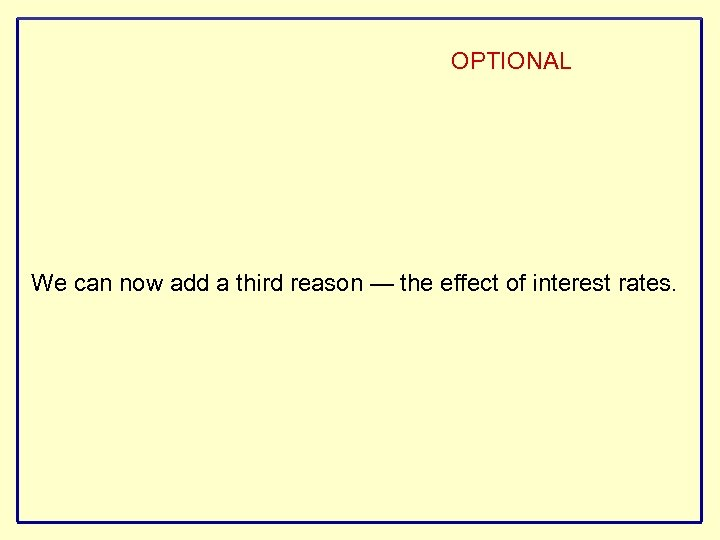OPTIONAL We can now add a third reason — the effect of interest rates.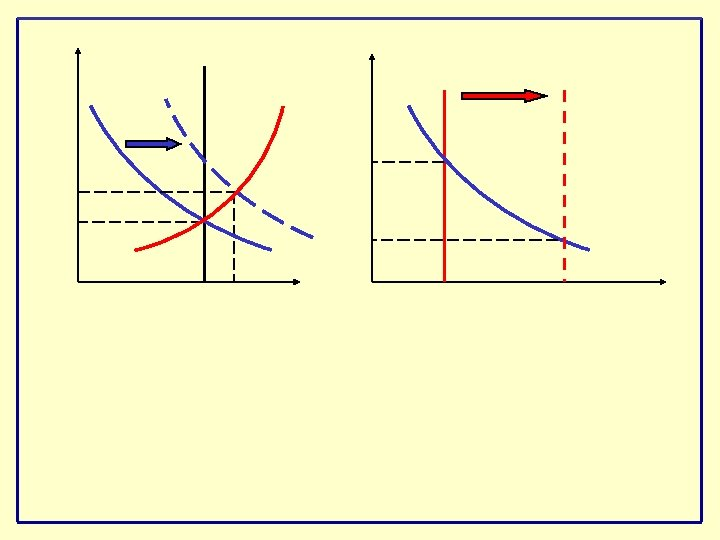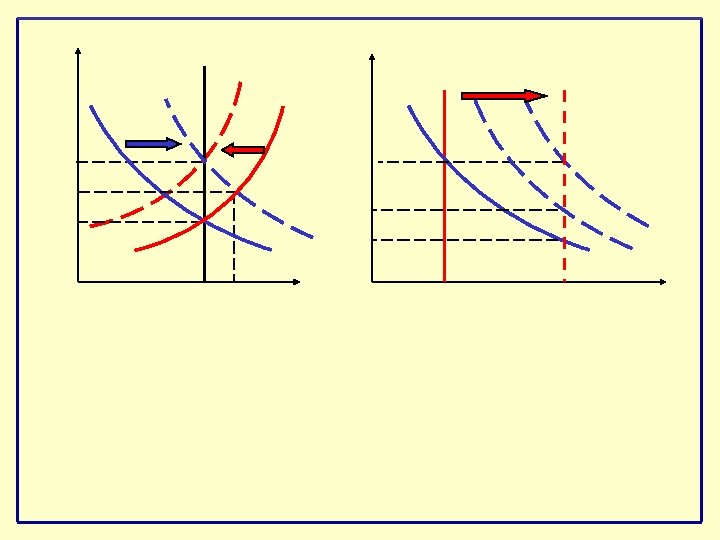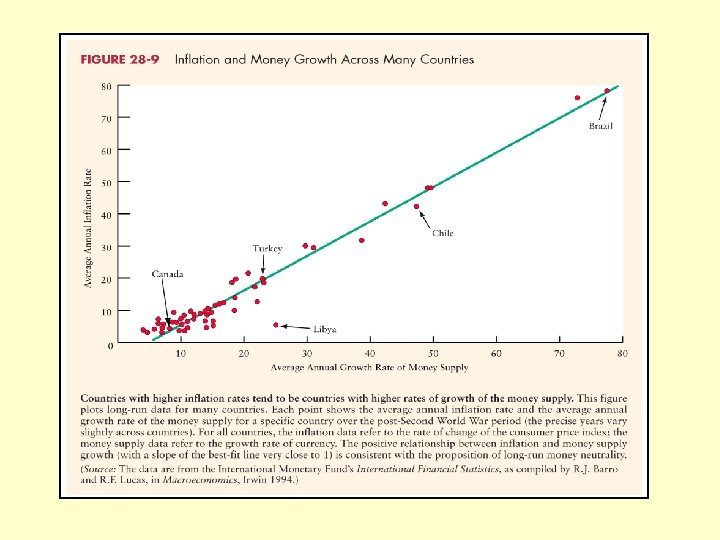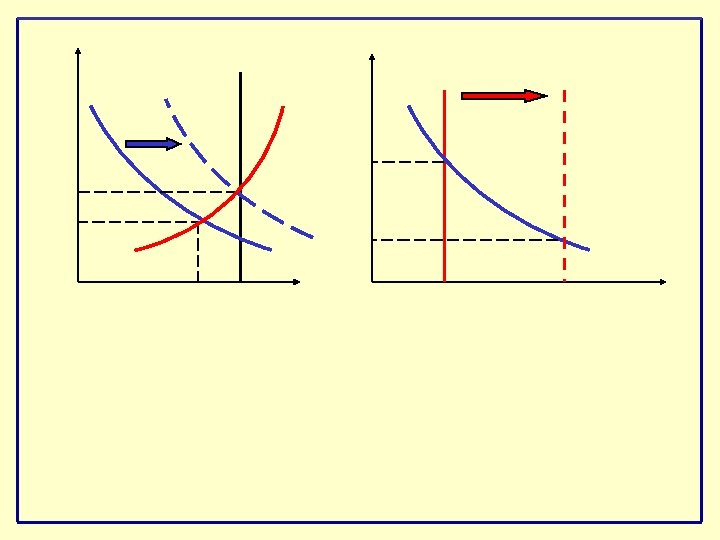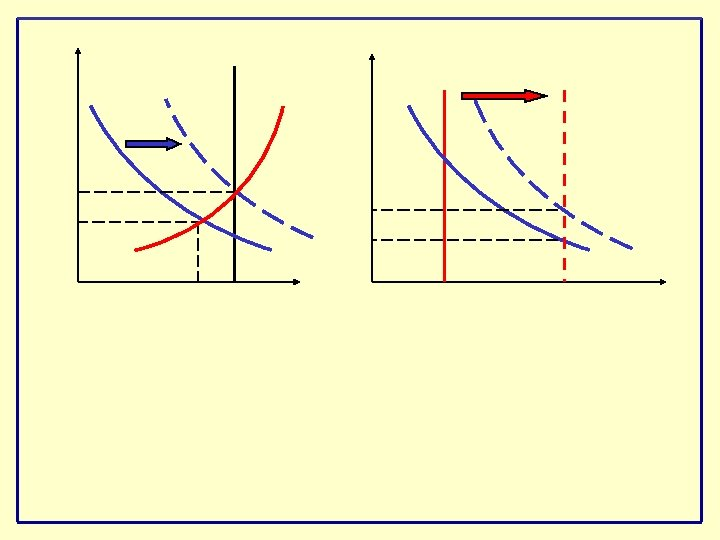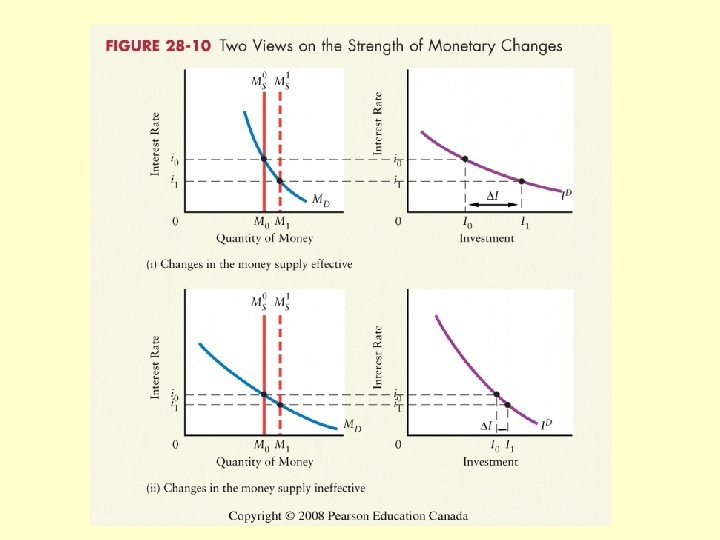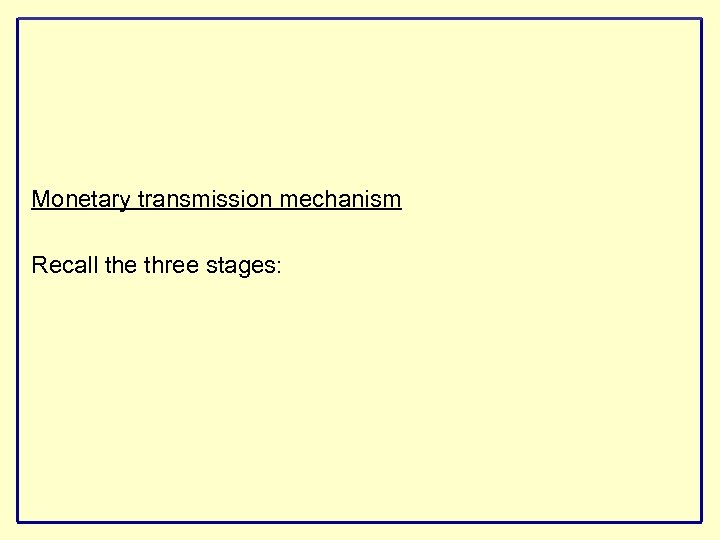Monetary transmission mechanism Recall the three stages: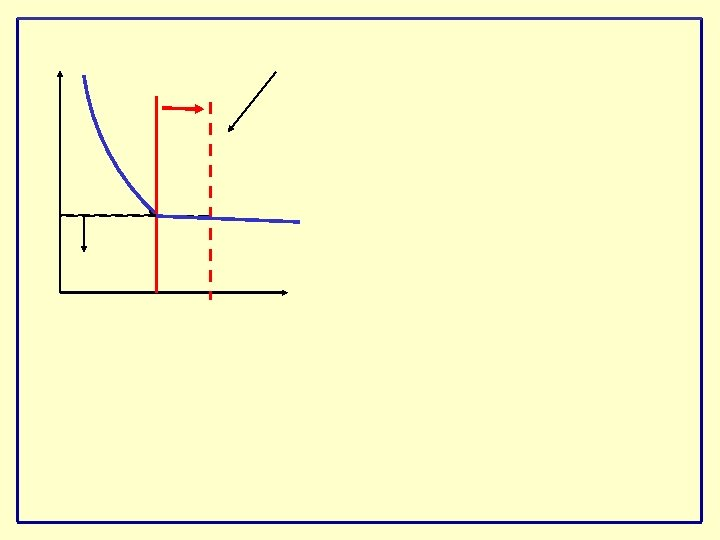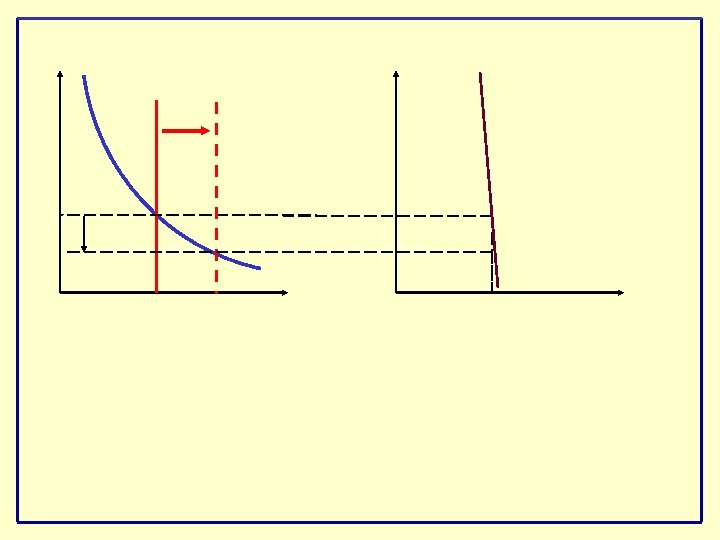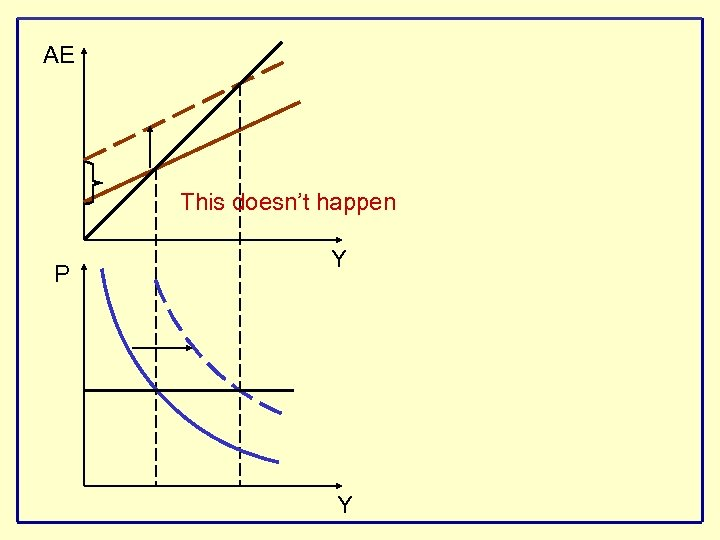AE This doesn’t happen P Y Y